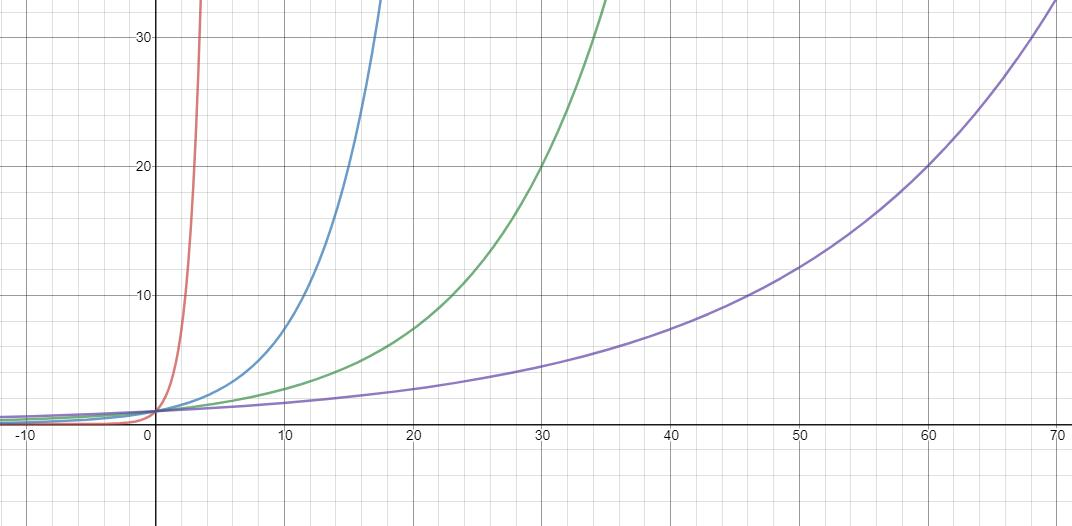# Google Bert 框架简单蒸馏实践

## Distilling the Knowledge in a Neural Network

方便，快捷，味道可口，快递给力 0.001 0.999



#### soft label 如何获得呢？

soft label 是大模型预测的概率分布，但大模型在训练时使用的是 hard label，而交叉熵损失函数会让模型的预测极力向正确的标签靠近，例如对于数据：

方便，快捷，味道可口，快递给力 1


s o f t − s o f t m a x ( z i ) = e x p ( z i / T ) ∑ j e x p ( z j / T ) soft-softmax(z_i) = \frac{exp(z_i / T)}{\sum_j exp(z_j / T)}• 红线：exp(x)
• 蓝线：exp(x/5)
• 绿线：exp(x/10)
• 紫线：exp(x/20)

T
10.99900.0090.001
100.70000.29000.010

#### 蒸馏目标

When the correct labels are known for all or some of the transfer set, this method can be significantly
improved by also training the distilled model to produce the correct labels. One way to do this is
to use the correct labels to modify the soft targets, but we found that a better way is to simply use
a weighted average of two different objective functions.

L K D = ( 1 − α ) H ( y , σ ( z S ) ) + α T 2 H ( σ ( z T / T ) , σ ( z S / T ) ) L_{KD} = (1 - \alpha)H(y, \sigma(z^S)) + \alpha T^2 H(\sigma(z^T / T), \sigma(z^S / T))

#### 蒸馏过程

In the simplest form of distillation, knowledge is transferred to the distilled model by training it on
a transfer set and using a soft target distribution for each case in the transfer set that is produced by
using the cumbersome model with a high temperature in its softmax. The same high temperature is
used when training the distilled model, but after it has been trained it uses a temperature of 1.

• 训练时：使用较大的 T 值来训练大模型，使大模型能够产生更平滑、均匀分布的 soft label，然后小模型使用相同的 T 值来学习 soft label。
• 推断时：在实际应用中，将 T 值调整回 1，让类别概率偏向正确类别。

## 代码实现

• 先训练大模型，然后使用训练后的大模型生成 soft label，然后将 soft label 添加到小模型的输入中。这种方式需要改动较多的代码，相当于将 soft label 一路从输入传递到最终的输出。
• 先训练大模型，然后将大模型导出成 saveModel。小模型在训练过程中调用大模型，从而生成相应的 soft label。本篇博客选择这一种方式。

### 实现细节

#### run_classifier.py

• temperature：蒸馏的温度值；
• do_distill：是否要进行蒸馏。
flags.DEFINE_integer("temperature", 1, "Temperature parameters in distillation operation.")
flags.DEFINE_bool("do_distill", False, "Whether to distill the model.")


with tf.variable_scope("loss"):
if is_training:
output_layer = tf.nn.dropout(output_layer, keep_prob=0.9)

logits = tf.matmul(output_layer, output_weights, transpose_b=True)
logits = tf.nn.bias_add(logits, output_bias)
one_hot_labels = tf.one_hot(labels, depth=num_labels, dtype=tf.float32)

if FLAGS.do_distill:
probabilities = tf.nn.softmax(logits / FLAGS.temperature, axis=-1)
log_probs = tf.nn.log_softmax(logits / FLAGS.temperature, axis=-1)
per_example_loss = -FLAGS.temperature ** 2 * tf.reduce_sum(one_hot_labels * log_probs, axis=-1)
else:
probabilities = tf.nn.softmax(logits, axis=-1)
log_probs = tf.nn.log_softmax(logits, axis=-1)
per_example_loss = -tf.reduce_sum(one_hot_labels * log_probs, axis=-1)
loss = tf.reduce_mean(per_example_loss)

return (loss, per_example_loss, logits, probabilities)


• train_teacher_model.sh
#! /bin/bash
export MODEL_DIR=model
export DATA_DIR=data

CUDA_VISIBLE_DEVICES=0 python bert/run_classifier.py \
--task_name=Emotion \
--do_train=true \
--do_eval=true \
--do_distill=true \
--data_dir=$DATA_DIR \ --vocab_file=$MODEL_DIR/vocab.txt \
--bert_config_file=$MODEL_DIR/bert_config.json \ --init_checkpoint=$MODEL_DIR/bert_model.ckpt \
--max_seq_length=128 \
--train_batch_size=32 \
--learning_rate=2e-5 \
--num_train_epochs=2.0 \
--output_dir=output/teacher \
--temperature=10


#### run_classifier_distill.py

• distill.sh
#! /bin/bash
export MODEL_DIR=model
export DATA_DIR=data

CUDA_VISIBLE_DEVICES=2 python bert/run_classifier.py \
--task_name=Emotion \
--do_train=true \
--do_eval=true \
--data_dir=$DATA_DIR \ --vocab_file=$MODEL_DIR/vocab.txt \
--bert_config_file=$MODEL_DIR/bert_config.json \ --init_checkpoint=$MODEL_DIR/bert_model.ckpt \
--max_seq_length=128 \
--train_batch_size=32 \
--learning_rate=2e-5 \
--num_train_epochs=2.0 \
--teacher_model=export/1591538997 \
--temperature=10 \
--alpha=0.5 \
--output_dir=output/distill


### 蒸馏结果

Bert_Base-0.60192
Bert_Base + FT0.91826920.91635
Bert_Small + FT0.91105770.91186
Bert_Small + FT + Distill0.91666670.91090

11-14657707-024062
01-22143
11-268790
04-082173
10-21258
11-2170
07-17894
11-05213
04-232674
10-241135
10-111349
06-301444
10-12323
07-011435
04-101387
02-2730
06-271万+
©️2020 CSDN 皮肤主题: 成长之路 设计师:Amelia_0503